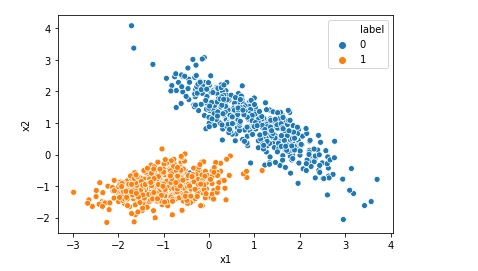# Creating Synthetic Data for Logistic Regression

Many a times we want to implement Logistic Regression on certain data but we do not find that kind of data online. In that case we can generate a synthetic data for our problem.

In this post we will see how to generate a typical synthetic data for a simple Logistic Regression.

Import the required libraries first.

``````import pandas as pd
import sklearn.datasets``````

Use the make classification class of sklearn

``````# Can set the number of rows, number of classes and number of features
data = sklearn.datasets.make_classification(n_samples=1000, n_classes=2,n_clusters_per_class=1, n_features=2,n_informative=2, n_redundant=0, n_repeated=0)``````

Create the data frame from above generated data.

``````x = data
y = data
df = pd.DataFrame(data)
df['label'] = data
1.asdefg says: